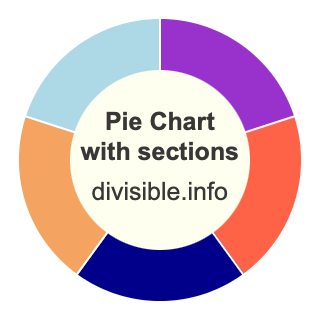Pie Chart with SectionsHere you can create a pie chart with sections and find out what each section is as a fraction and as a percentage.

Each section of the pie chart comes with a different color, and we explain how we calculated what each section is as a fraction and what each section is as a percentage.

Please enter how many sections you want to divide a pie chart into.

Pie chart with  sections

Below are some examples of pie charts that we have divided into sections.

Pie chart with 5 sections

Pie chart with 6 sections

Pie chart with 3 sections

Pie chart with 8 sections

Pie chart with 10 sections